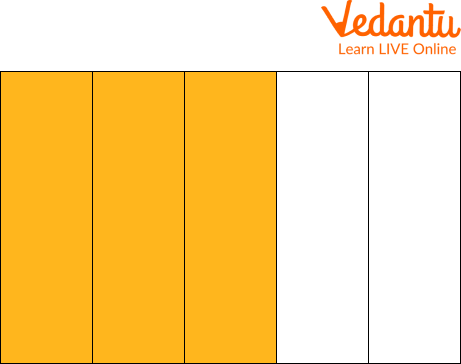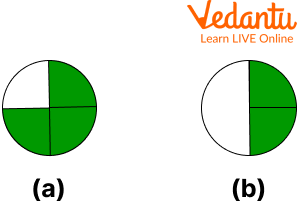Courses
Courses for Kids
Free study material
Free LIVE classes
More

# Parts of FractionLIVE
Join Vedantu’s FREE Mastercalss

## Introduction to Fractions

Fractions are important because they tell you what portion of a whole you need, have, or want. In this, we will discuss the identification of the parts of a fraction. We know fraction means part of something. Fraction tells us about how many parts a whole has been divided into and the part which is considered out of the whole.

We also know that the numbers \$0,1,2,3\$, are called whole numbers and the numbers \$\dfrac{1}{2}, \dfrac{1}{3}, \dfrac{1}{4}, \dfrac{1}{5}\$, are called fractional numbers.

## Parts of Fraction

Every fraction number has two numbers separated by a ‘-‘ (dash) called Fractional bar which means divided.

Numerator is the number above the bar and it tells us as to how many parts have been considered out of the total equally divided parts.

Denominator is the number below the bar and it describes us as in how many parts a whole has been divided.Parts of Fractions

## What is the Fraction of the Shaded Area?Fraction

This rectangle is considered as a whole. It is divided into 8 parts and this 8 represents the denominator of a fraction.

Three parts are shaded. This 3 represents the numerator.

So, the fraction of the shaded part is \$\dfrac{3}{8}\$

## Identify the Fraction

Students represent a simple fraction by coloring in blocks. The number of blocks is represented by the denominator, and the numerator represents the number of blocks to be colored in.

For example,

If we wanna find the fraction of \$\dfrac{3}{5}\$Fraction

Hence, the shaded region represents the parts from the whole.

## Solved Examples

What fraction of each shape is colored?Question

(a)

1. \$\dfrac{1}{4}\$

2. \$\dfrac{1}{2}\$

3. \$\dfrac{3}{4}\$

4. \$\dfrac{5}{3}\$

Ans. b) \$\dfrac{1}{2}\$

(b)

1. \$\dfrac{1}{3}\$

2. \$\dfrac{5}{2}\$

3. \$\dfrac{1}{3}\$

4. \$\dfrac{5}{3}\$

Ans. c) \$\dfrac{1}{3}\$

(c)

1. \$\dfrac{1}{3}\$

2. \$\dfrac{2}{5}\$

3. \$\dfrac{5}{3}\$

4. \$\dfrac{1}{6}\$

Ans. d) \$\dfrac{1}{6}\$

(d)

1. \$\dfrac{1}{4}\$

2. \$\dfrac{1}{2}\$

3. \$\dfrac{3}{4}\$

4. \$\dfrac{5}{3}\$

Ans. a) \$\dfrac{1}{4}\$

## Practice Questions

What fraction of each shape is colored?(a)

1. \$\dfrac{1}{4}\$

2. \$\dfrac{1}{2}\$

3. \$\dfrac{3}{4}\$

4. \$\dfrac{5}{3}\$

Ans. c) \$\dfrac{3}{4}\$

(b)

1. \$\dfrac{1}{4}\$

2. \$\dfrac{2}{4}\$

3. \$\dfrac{4}{3}\$

4. \$\dfrac{5}{3}\$

Ans. b) \$\dfrac{2}{4}\$

## Summary

In this article, we learned about fractions, how a fraction is a part of something and shows how many parts the whole has been divided into. The two numbers are separated by the fractional bar and the number above the bar is called the numerator and the number below the bar is called the denominator.

We also learned about how we can figure out fractions by looking at the shaded area when it comes to solving questions and figuring out the fractions. How we can solve fractional problems like addition, subtraction, multiplication, and division etc.

Last updated date: 26th Sep 2023
Total views: 68.4k
Views today: 1.68k

## FAQs on Parts of Fraction

1. What is a fraction in Mathematics?

A fraction is a numerical value that is a part of a whole. It is shown by dividing a whole into a number of parts. For example, \$\dfrac{1}{2}\$ represents half of a whole number or a thing.

2. What are the three types of fractions?

There are three types of fractions: Proper fractions, Improper fractions and Mixed fractions.

• Proper fraction: Fraction where numerator is lesser than the denominator.

• Improper fraction:Fraction where numerator is greater than or equal to the denominator.

• Mixed fraction: a fraction represented with its quotient and remainder.

3. What is \$\dfrac{3}{4}\$ in decimals?

\$\dfrac{3}{4}\$ is equal to 0.75 in decimals.